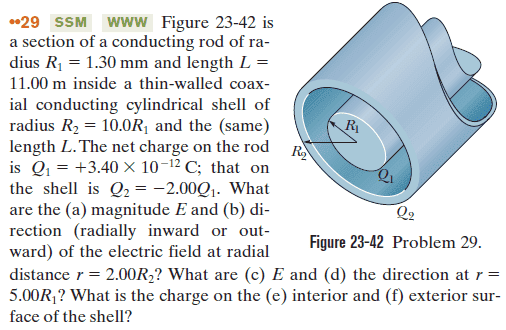# Applying Gauss’ Law

yohanes vianei

## Homework StatementE.dA = qencl/ϵ0

## The Attempt at a Solution

(a) magnitude E

we use

E.dA = qencl/ϵ0

For a cylinder:

E.dA = E(2πrL), then

qencl/ϵ0 = E(2πrL)

E = qencl/(2πrLϵ0)

E = λ/(2πrϵ0); WITH λ = qencl/L

(a) the magnitude E,

qencl = Q1 + Q2 , then

E = (Q1 + Q2)/(2πrLϵ0)

Because:

· Q1 + Q2 = +3.40 x 10─12 C +( ─2 x 3.40 x 10─12 C) = ─3.40 x 10─12 C,

· Radius, r = 2.00R2 = 2.00 (10R1) = 20 x 1.3 mm = 26 x 10─3 m

· length L = 11.00 m

then,

E = (─3.40 x 10─12 C)/(2π x 26 x 10─3 m x 11.00 m x 8.85 x10-12 C2/Nm2)

E = 0.214 N/C

(b) direction (radially inward or outward) of the electric field at radial distance r = 2.00R2 is radially inward since qencl < 0

(c) E for r = 5.00R1 = 5.00 x 1.3 mm = 6.5 x 10─3 m, and qencl = Q1 = +3.40 x 10─12 C, then

E = (+3.40 x 10─12 C)/(2π x 6.5 x 10─3 m x 11.00 m x 8.85 x10-12 C2/Nm2)

E = 0.855 N/C

(d) the direction at r = 5.00R1 is radially outward since qencl > 0

(e) the charge on the interior is

Qinner = ─ Q1 = ─3.40 x 10─12 C

(f) the charge on the exterior surface of the shell is

Qouter = Q2 ─ Qinner = (─2 x 3.40 x 10─12 C) ─ (─3.40 x 10─12 C)

Qouter = ─3.40 x 10─12 C

[/B]

#### Attachments

•